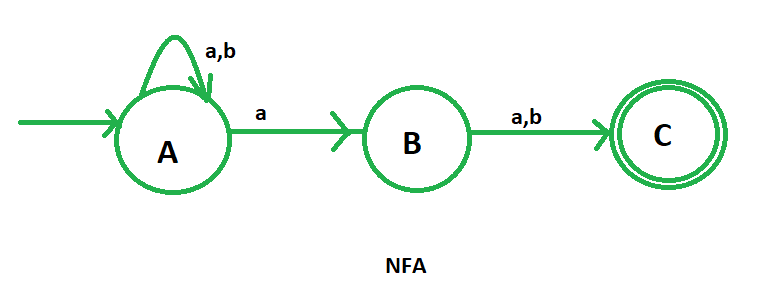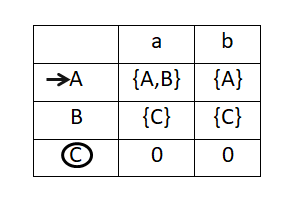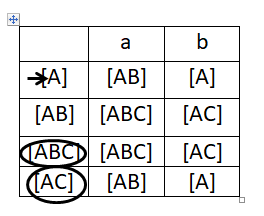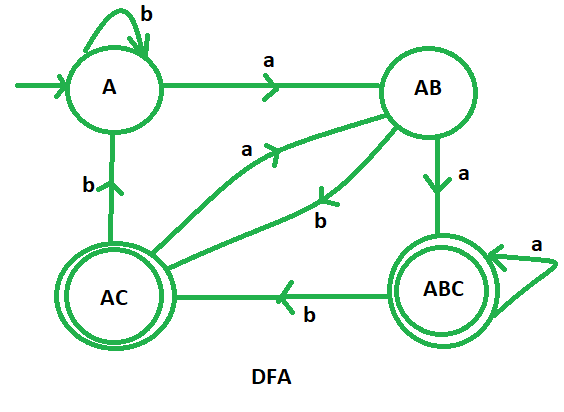# DFA of a string in which 2nd symbol from RHS is ‘a’

Prerequisite – Finite Automata Introduction
Problem – Draw deterministic finite automata (DFA) of the language containing the set of all strings over {a, b} in which 2nd symbol from RHS is ‘a’.

The strings in which 2nd last symbol is “a” are:

```aa, ab, aab, aaa, aabbaa, bbbab etc
```

For example:

```INPUT : baba
OUTPUT: NOT ACCEPTED

INPUT: aaab
OUTPUT: ACCEPTED
```

Constructing the DFA of the given problem directly is very complicated. So, here we are going to design the non-deterministic finite automata (NFA) and then convert it to the deterministic finite automata (DFA).

The NFA of the language containing all the strings in which 2nd symbol from the RHS is “a” is:Here, A is the initial state and C is the final state.
Now, we are going to construct the state transition table of the above NFA.After that we will draw the state transition table of DFA using subset configuration on the state transition table of NFA. We will mention all the possible transition for a and b.Now it’s become very easy to draw the DFA with the help of its transition table. In this DFA, we have four different states A, AB, ABC and AC, where ABC and AC are the final states and A is the initial state of the DFA.This is our required DFA of the language containing the set of all strings over {a, b} in which 2nd symbol from RHS is ‘a’.

### Transition table:

 STATES INPUT (a) INPUT (b) —> A (initial state) AB A AB ABC* (final state) AC* (final state) AC* (final state) AB A ABC* (final state) ABC* (final state) AC* (final state)

### Python Implementation:

 `def` `stateA(n): ` `    ``#if length found 0  ` `    ``#print not accepted ` `    ``if` `(``len``(n)``=``=``0``): ` `        ``print``(``"string not accepted"``) ` `    ``else``:     ` `         `  `        ``#if at index 0  ` `        ``#'a' found call  ` `        ``#function stateAB  ` `        ``if``(n[``0``]``=``=``'a'``): ` `            ``stateAB(n[``1``:]) ` `             `  `        ``#else if 'b' found  ` `        ``#call function A.     ` `        ``elif` `(n[``0``]``=``=``'b'``): ` `            ``stateA(n[``1``:]) ` `         `  `def` `stateAB(n): ` `    ``#if length found 0  ` `    ``#print not accepted ` `    ``if` `(``len``(n)``=``=``0``): ` `        ``print``(``"string not accepted"``) ` `    ``else``: ` `         `  `        ``#if at index 0  ` `        ``#'a' found call  ` `        ``#function stateABC ` `        ``if``(n[``0``]``=``=``'a'``): ` `            ``stateABC(n[``1``:]) ` `             `  `        ``#else if 'b' found  ` `        ``#call function AC.      ` `        ``elif` `(n[``0``]``=``=``'b'``): ` `            ``stateAC(n[``1``:])  ` `         `  `def` `stateABC(n): ` `    ``#if length found 0  ` `    ``#print accepted ` `    ``if` `(``len``(n)``=``=``0``): ` `        ``print``(``"string accepted"``) ` `    ``else``: ` `         `  `        ``#if at index 0  ` `        ``#'a' found call  ` `        ``#function stateABC ` `        ``if``(n[``0``]``=``=``'a'``): ` `            ``stateABC(n[``1``:]) ` `             `  `        ``#else if 'b' found  ` `        ``#call function AC.      ` `        ``elif` `(n[``0``]``=``=``'b'``): ` `            ``stateAC(n[``1``:]) ` `         `  `def` `stateAC(n): ` `    ``#if length found 0  ` `    ``#print accepted ` `    ``if` `(``len``(n)``=``=``0``): ` `        ``print``(``"string accepted"``) ` `    ``else``: ` `        ``#if at index 0  ` `        ``#'a' found call  ` `        ``#function stateAB ` `        ``if``(n[``0``]``=``=``'a'``): ` `            ``stateAB(n[``1``:]) ` `             `  `        ``#else if 'b' found  ` `        ``#call function A.      ` `        ``elif` `(n[``0``]``=``=``'b'``): ` `            ``stateA(n[``1``:])         ` `   `  ` `  `#take string input ` `n``=``input``() ` ` `  `#call stateA ` `#to check the input ` `stateA(n) `

Attention reader! Don’t stop learning now. Get hold of all the important CS Theory concepts for SDE interviews with the CS Theory Course at a student-friendly price and become industry ready.

My Personal Notes arrow_drop_upCheck out this Author's contributed articles.

If you like GeeksforGeeks and would like to contribute, you can also write an article using contribute.geeksforgeeks.org or mail your article to contribute@geeksforgeeks.org. See your article appearing on the GeeksforGeeks main page and help other Geeks.

Please Improve this article if you find anything incorrect by clicking on the "Improve Article" button below.

Improved By : _mridul_bhardwaj_